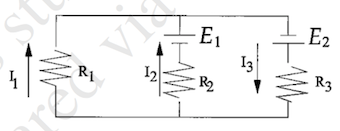# Problem: The following equation is true for the right loop if the circuit. E2 – I3R3 – I2R2 – E1 = 0 –E2 – I3R3 – I2R2 – E1 = 0 E2 – I3R3 – I2R2 + E1 = 0 E2 + I3R3 + I2R2 – E1 = 0 None of the above

###### Problem Details

The following equation is true for the right loop if the circuit.

1. E2 – I3R3 – I2R2 – E1 = 0
2. –E2 – I3R3 – I2R2 – E1 = 0
3. E2 – I3R3 – I2R2 + E1 = 0
4. E2 + I3R3 + I2R2 – E1 = 0
5. None of the above